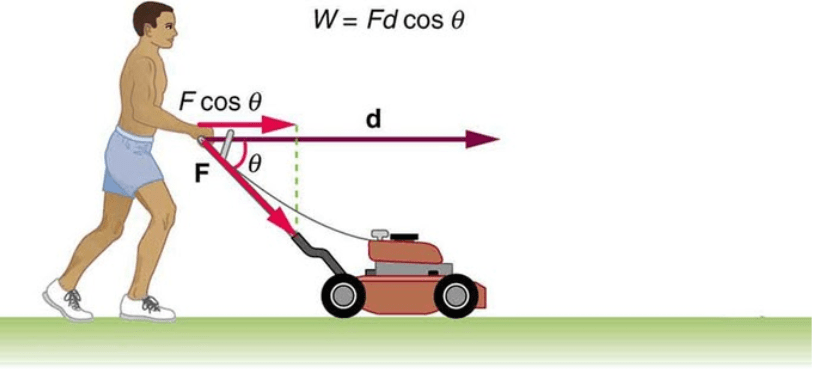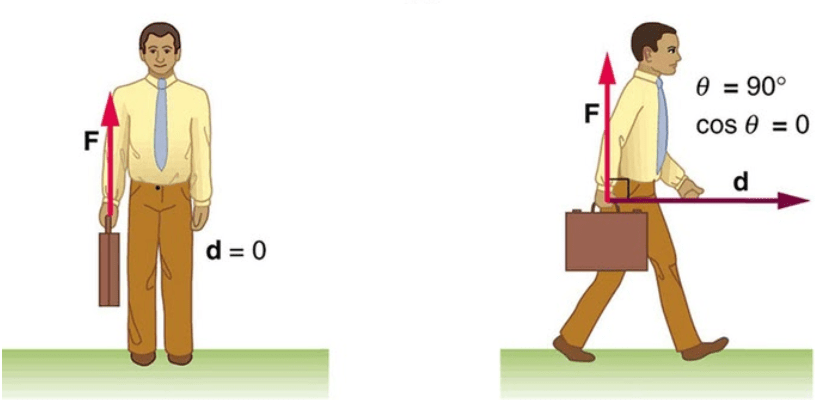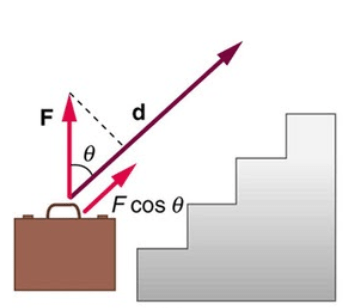Complimentary 1-hour tutoring consultation

MCAT Content / Work / Work Done By A Constant Force W Fd Cos0

### Work done by a constant force: W = Fd cosθ

Topic: Work

The work done on a system by a constant force is the product of the component of the force in the direction of motion times the distance through which the force acts.W is work, F is the magnitude of the force on the system, d is the magnitude of the displacement of the system, and θ is the angle between the force vector F and the displacement vector d.

Examples of work:

1. The work done by the force F on this lawnmower is Fdcosθ.2. A person holding a briefcase does no work on it because there is no motion. No energy is transferred to or from the briefcase. The person moving the briefcase horizontally at a constant speed does no work on it and transfers no energy to it.3. Work is done on the briefcase by carrying it upstairs at a constant speed because there is necessarily a component of force F in the direction of the motion. Energy is transferred to the briefcase and could, in turn, be used to do work.The work can be calculated as the area under the force-displacement graph. This is true for work done by a constant force (like the examples shown here), as well as work done by a variable force. Work done by an elastic force, for example, follows the equation W = ksx2/2, where ks is the spring constant of the force and x is the displacement. Work can also be calculated in these systems from force-displacement graphs.

Practice Questions

Coronary heart disease and blood pressure

MCAT Official Prep (AAMC)

Physics Online Flashcards Question 10

Physics Question Pack Passage 7 Question 45

Physics Question Pack Question 57

Physics Question Pack Question 74

Physics Question Pack Passage 13 Question 79

Physics Question Pack Passage 13 Question 82

Official Guide C/P Section Passage 2 Question 7

Practice Exam 3 B/B Section Passage 1 Question 1

Practice Exam 3 B/B Section Passage 1 Question 4

Key Points

• Work is the transfer of energy by a force acting on an object as it is displaced.

• The work done by a force is zero if the displacement is either zero or perpendicular to the force.

• The work done is positive if the force and displacement have the same direction, and the work done is negative if they have opposite direction.

Key Terms

Energy: A quantity that denotes the ability to do work and is measured in a unit dimensioned in mass × distance²/time² (ML²/T²) or the equivalent

Force-displacement graph: A graph of the force applied on the y-axis, and distance moved on the x-axis. The area under the curve between two points is the work done.

Billing Information